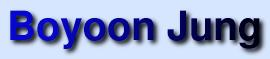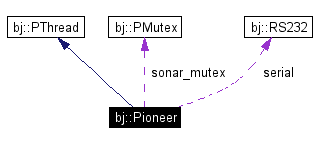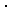Boyoon Jung's Library Manual

# bj::Pioneer Class Reference [Robot Programming]

A device driver for robots using P2OS. More...

`#include <pioneer.h>`

Inherits bj::PThread.

Inheritance diagram for bj::Pioneer:[legend]
Collaboration diagram for bj::Pioneer:[legend]
List of all members.

## Public Member Functions

Pioneer (char *device=0, speed_t baudrate=B9600)
A constructor.
~Pioneer (void)
A destructor.
bool open (char *device, speed_t baudrate=B9600)
Open a serial port for communication to SICK laser device.
bool close (void)
Close the serial port.
bool enableMotor (bool enable=true)
Enable/disable motors.
bool disableMotor (void)
Disable motors.
bool setHeading (double head)
Set an absolute heading to rotate.
bool setDistance (double distance)
Set an absolute distance to travel.
bool setAngularVelocity (double vel)
Set an angular velocity.
bool setLinearVelocity (double vel)
Set a linear velocity.
bool setControlPoint (double dchead)
Set a control point.
bool setDifferentialHeading (double dhead)
Set a differential heading from the current control point (DHEAD).
bool setIndependentVeolocities (double left, double right)
Set independent wheel velocities.
bool setMaxAngularVelocity (double vel)
Set the maximum angular velocity used for Colbert move command speed.
bool setMaxLinearVelocity (double vel)
Set the maximum linear velocity used for Colbert move command speed.
bool setAngularAccel (double accel)
Set an angular acceleration/deceleration to achieve setpoint.
bool setLinearAccel (double accel)
Set an linear acceleration/deceleration to achieve setpoint.
bool setAngularPGain (int p)
Set the proportional gain for angular velocity control.
bool setAngularIGain (int i)
Set the integral gain for angular velocity control.
bool setAngularDGain (int d)
Set the differential gain for angular velocity control.
bool setAngularPIDGain (int p, int i, int d)
Set the PID gains for angular velocity control.
bool setLinearPGain (int p)
Set the proportional gain for linear velocity control.
bool setLinearIGain (int i)
Set the integral gain for linear velocity control.
bool setLinearDGain (int d)
Set the differential gain for linear velocity control.
bool setLinearPIDGain (int p, int i, int d)
Set the PID gains for linear velocity control.
bool resetOdometer (void)
Reset odometer.
bool stop (void)
Stop a robot.
bool emergentStop (void)
Emergent stop.
bool enableSonar (bool enable=true)
Enable/disable sonar transducers.
bool disableSonar (void)
Disable sonar transducers.
bool setDigitalOutput (int port, bool set)
Set/unset a digital output port.
bool selectAnalogPort (int port)
Select an analog port.
pioneer_t type (void)
Return the pioneer type.
char * name (void)
Return the name of a robot.
bool isMotorEnabled (void)
Check if motors are enabled.
bool isMoving (void)
Check if a robot is moving.
double x (void)
Return the current X position in odometry coordinates.
double y (void)
Return the current Y position in odometry coordinates.
void position (double &x, double &y)
Return the current position in odometry coordinates.
double heading (void)
Return the current heading in odometry coordinates.
void pose (double &x, double &y, double &h)
Return the current pose in odometry coordinates.
float leftVelocity (void)
Return the current velocity of the left wheel.
float rightVelocity (void)
Return the current velocity of the right wheel.
void velocity (double &l, double &r)
Return the current velocity of the wheels.
float battery (void)
Return the current battery level in voltages.
bool isLeftStall (void)
Check if the left wheel is stalled.
bool isRightStall (void)
Check if the right wheel is stalled.
bool isStall (void)
Check if any of the wheels is stalled.
bool bumper (int n)
Check if the specific bumper is pressed.
void bumpers (bool b[])
Return the all bummer status.
double controlPoint (void)
Return the current control point.
bool isSonarEnabled (void)
Check if the sonar transducers are enabled.
int numSonars (void)
Return the number of sonar transducers connected.
int sonars (float s[])
Retrieve the sonar range values.
const double * sonarPose (int i)
Return the pose of the specific sonar transducer in the robot body's coordinates.
double sonarXPosition (int i)
Return the X position of the specific sonar transducer in the robot body's coordinates.
double sonarYPosition (int i)
Return the Y position of the specific sonar transducer in the robot body's coordinates.
double sonarHeading (int i)
Return the heading of the specific sonar transducer in the robot body's coordinates.
unsigned short compass (void)
Return the current absolute heading.
int analogPort (void)
Return the analog port number selected.
unsigned char analogInput (int p=-1)
Return the input value of the currently selected analog port.
bool digitalInput (int n)
Return the value of a specific digial input port.
bool digitalOutput (int n)
Return the value of a specific digial output port.

## Protected Member Functions

int read_packet (unsigned char *data)
Read a packet from a serial port.
bool send_command (unsigned char cmd)
Send a command.
bool send_command (unsigned char cmd, int arg)
Send a command with an integer argument.
bool send_command (unsigned char cmd, char *arg, int len)
Send a command with a string argument.
void store_robot_config (unsigned char *data)
Store a robot configuration info.
void * run (void)
Update the local info periodically.

## Static Protected Member Functions

double get_time (void)
Get the current time in floating-point format.
int create_checksum (unsigned char *data, int len)
Create a checksum.
void append_checksum (unsigned char *data, int len)
Create a checksum and append it to the end of data.
int bytes2int (unsigned char *bytes)
Convert a byte string to a 2-byte signed integer.
int bytes2uint (unsigned char *bytes)
Convert a byte string to a 2-byte unsigned integer.
void int2bytes (int i, unsigned char *bytes)
Convert a 2-byte integer to a byte string.
int bytes2checksum (unsigned char *bytes)
Convert a byte string to a checksum.
void checksum2bytes (int cs, unsigned char *bytes)
Convert a checksum to a byte string.

## Detailed Description

A device driver for robots using P2OS.

The coordinate system for robot control are:

• linear distance / velocity / acceleration
• unit: meter
• (+) forward
• (-) reverse
• angular distance / velocity / acceleration
• unit: radian
• (+) counter-clockwise
• (-) clockwise

There are some devices that are not supported yet:

• gripper
• arm device

## Constructor & Destructor Documentation

 bj::Pioneer::Pioneer ( char * device = `0`, speed_t baudrate = `B9600` )
 A constructor.

 bj::Pioneer::~Pioneer ( void )
 A destructor.

## Member Function Documentation

 unsigned char bj::Pioneer::analogInput ( int p = `-1` ) ` [inline]`
 Return the input value of the currently selected analog port.

 int bj::Pioneer::analogPort ( void ) ` [inline]`
 Return the analog port number selected.

 void bj::Pioneer::append_checksum ( unsigned char * data, int len ) ` [inline, static, protected]`
 Create a checksum and append it to the end of data.

 float bj::Pioneer::battery ( void ) ` [inline]`
 Return the current battery level in voltages.

 bool bj::Pioneer::bumper ( int n ) ` [inline]`
 Check if the specific bumper is pressed.

 void bj::Pioneer::bumpers ( bool b[] ) ` [inline]`
 Return the all bummer status.

 int bj::Pioneer::bytes2checksum ( unsigned char * bytes ) ` [inline, static, protected]`
 Convert a byte string to a checksum.

 int bj::Pioneer::bytes2int ( unsigned char * bytes ) ` [inline, static, protected]`
 Convert a byte string to a 2-byte signed integer.

 int bj::Pioneer::bytes2uint ( unsigned char * bytes ) ` [inline, static, protected]`
 Convert a byte string to a 2-byte unsigned integer.

 void bj::Pioneer::checksum2bytes ( int cs, unsigned char * bytes ) ` [inline, static, protected]`
 Convert a checksum to a byte string.

 bool bj::Pioneer::close ( void )
 Close the serial port.

 unsigned short bj::Pioneer::compass ( void ) ` [inline]`
 Return the current absolute heading. Returns:The current absolute heading in degrees.

 double bj::Pioneer::controlPoint ( void ) ` [inline]`
 Return the current control point. Returns:The current control point in radians.

 int bj::Pioneer::create_checksum ( unsigned char * data, int len ) ` [inline, static, protected]`
 Create a checksum.

 bool bj::Pioneer::digitalInput ( int n ) ` [inline]`
 Return the value of a specific digial input port.

 bool bj::Pioneer::digitalOutput ( int n ) ` [inline]`
 Return the value of a specific digial output port.

 bool bj::Pioneer::disableMotor ( void ) ` [inline]`
 Disable motors.

 bool bj::Pioneer::disableSonar ( void ) ` [inline]`
 Disable sonar transducers.

 bool bj::Pioneer::emergentStop ( void ) ` [inline]`
 Emergent stop.

 bool bj::Pioneer::enableMotor ( bool enable = `true` ) ` [inline]`
 Enable/disable motors.

 bool bj::Pioneer::enableSonar ( bool enable = `true` ) ` [inline]`
 Enable/disable sonar transducers.

 double bj::Pioneer::get_time ( void ) ` [inline, static, protected]`
 Get the current time in floating-point format.

 double bj::Pioneer::heading ( void ) ` [inline]`
 Return the current heading in odometry coordinates. Returns:The current heading in odometry coordinates in radians.

 void bj::Pioneer::int2bytes ( int i, unsigned char * bytes ) ` [inline, static, protected]`
 Convert a 2-byte integer to a byte string.

 bool bj::Pioneer::isLeftStall ( void ) ` [inline]`
 Check if the left wheel is stalled.

 bool bj::Pioneer::isMotorEnabled ( void ) ` [inline]`
 Check if motors are enabled.

 bool bj::Pioneer::isMoving ( void ) ` [inline]`
 Check if a robot is moving.

 bool bj::Pioneer::isRightStall ( void ) ` [inline]`
 Check if the right wheel is stalled.

 bool bj::Pioneer::isSonarEnabled ( void ) ` [inline]`
 Check if the sonar transducers are enabled.

 bool bj::Pioneer::isStall ( void ) ` [inline]`
 Check if any of the wheels is stalled.

 float bj::Pioneer::leftVelocity ( void ) ` [inline]`
 Return the current velocity of the left wheel. Returns:The current velocity of the left wheel in meters per second.

 char* bj::Pioneer::name ( void ) ` [inline]`
 Return the name of a robot.

 int bj::Pioneer::numSonars ( void ) ` [inline]`
 Return the number of sonar transducers connected.

 bool bj::Pioneer::open ( char * device, speed_t baudrate = `B9600` )
 Open a serial port for communication to SICK laser device.

 void bj::Pioneer::pose ( double & x, double & y, double & h ) ` [inline]`

Return the current pose in odometry coordinates.

Parameters:
 [out] x The current X position in odometry coordinates in meters. [out] y The current Y position in odometry coordinates in meters. [out] h The current heading in odometry coordinates in radians.

 void bj::Pioneer::position ( double & x, double & y ) ` [inline]`

Return the current position in odometry coordinates.

Parameters:
 [out] x The current X position in odometry coordinates in meters. [out] y The current Y position in odometry coordinates in meters.

 int bj::Pioneer::read_packet ( unsigned char * data ) ` [protected]`
 Read a packet from a serial port.

 bool bj::Pioneer::resetOdometer ( void ) ` [inline]`
 Reset odometer.

 float bj::Pioneer::rightVelocity ( void ) ` [inline]`
 Return the current velocity of the right wheel. Returns:The current velocity of the right wheel in meters per second.

 void* bj::Pioneer::run ( void ) ` [protected, virtual]`
 Update the local info periodically. Implements bj::PThread.

 bool bj::Pioneer::selectAnalogPort ( int port ) ` [inline]`
 Select an analog port.

 bool bj::Pioneer::send_command ( unsigned char cmd, char * arg, int len ) ` [inline, protected]`
 Send a command with a string argument.

 bool bj::Pioneer::send_command ( unsigned char cmd, int arg ) ` [inline, protected]`
 Send a command with an integer argument.

 bool bj::Pioneer::send_command ( unsigned char cmd ) ` [inline, protected]`
 Send a command.

 bool bj::Pioneer::setAngularAccel ( double accel ) ` [inline]`

Set an angular acceleration/deceleration to achieve setpoint.

Parameters:
 accel Angular acceleration/deceleration in meters per second square. If positive, it sets the angular acceleration, and if negative, it sets the angular deceleration.

 bool bj::Pioneer::setAngularDGain ( int d ) ` [inline]`
 Set the differential gain for angular velocity control.

 bool bj::Pioneer::setAngularIGain ( int i ) ` [inline]`
 Set the integral gain for angular velocity control.

 bool bj::Pioneer::setAngularPGain ( int p ) ` [inline]`
 Set the proportional gain for angular velocity control.

 bool bj::Pioneer::setAngularPIDGain ( int p, int i, int d ) ` [inline]`
 Set the PID gains for angular velocity control.

 bool bj::Pioneer::setAngularVelocity ( double vel ) ` [inline]`

Set an angular velocity.

Parameters:
 vel Angular velocity in radians.

 bool bj::Pioneer::setControlPoint ( double dchead ) ` [inline]`

Set a control point.

Parameters:
 dchead Control point in radians.

 bool bj::Pioneer::setDifferentialHeading ( double dhead ) ` [inline]`

Set a differential heading from the current control point (DHEAD).

Parameters:
 dhead Differential heading in radians.

 bool bj::Pioneer::setDigitalOutput ( int port, bool set ) ` [inline]`
 Set/unset a digital output port.

 bool bj::Pioneer::setDistance ( double distance ) ` [inline]`

Set an absolute distance to travel.

Parameters:
 distance Absolute distance to travel in meters.

 bool bj::Pioneer::setHeading ( double head ) ` [inline]`

Set an absolute heading to rotate.

Parameters:
 head Absolute heading to rotate in radians.

 bool bj::Pioneer::setIndependentVeolocities ( double left, double right ) ` [inline]`

Set independent wheel velocities.

Parameters:
 left Desired velocity of the left wheel in meters. right Desired velocity of the right wheel in meters.

 bool bj::Pioneer::setLinearAccel ( double accel ) ` [inline]`

Set an linear acceleration/deceleration to achieve setpoint.

Parameters:
 accel Linear acceleration/deceleration in meters per second square. If positive, it sets the linear acceleration, and if negative, it sets the linear deceleration.

 bool bj::Pioneer::setLinearDGain ( int d ) ` [inline]`
 Set the differential gain for linear velocity control.

 bool bj::Pioneer::setLinearIGain ( int i ) ` [inline]`
 Set the integral gain for linear velocity control.

 bool bj::Pioneer::setLinearPGain ( int p ) ` [inline]`
 Set the proportional gain for linear velocity control.

 bool bj::Pioneer::setLinearPIDGain ( int p, int i, int d ) ` [inline]`
 Set the PID gains for linear velocity control.

 bool bj::Pioneer::setLinearVelocity ( double vel ) ` [inline]`

Set a linear velocity.

Parameters:
 vel Linear velocity in meters.

 bool bj::Pioneer::setMaxAngularVelocity ( double vel ) ` [inline]`

Set the maximum angular velocity used for Colbert move command speed.

Parameters:
 vel Maximum angular velocity in radians.

 bool bj::Pioneer::setMaxLinearVelocity ( double vel ) ` [inline]`

Set the maximum linear velocity used for Colbert move command speed.

Parameters:
 vel Maximum linear velocity in meters.

 double bj::Pioneer::sonarHeading ( int i ) ` [inline]`
 Return the heading of the specific sonar transducer in the robot body's coordinates. Returns:The Y position of the specific sonar transducer in the robot body's coordinates in meters.

 const double* bj::Pioneer::sonarPose ( int i ) ` [inline]`
 Return the pose of the specific sonar transducer in the robot body's coordinates. Returns:The pose of the specific sonar transducer, which is an array of 3 double values. The first and the second values are the X and Y positions in meters, and the third one is the heading angule in radians.

 int bj::Pioneer::sonars ( float s[] ) ` [inline]`
 Retrieve the sonar range values.

 double bj::Pioneer::sonarXPosition ( int i ) ` [inline]`
 Return the X position of the specific sonar transducer in the robot body's coordinates. Returns:The X position of the specific sonar transducer in the robot body's coordinates in meters.

 double bj::Pioneer::sonarYPosition ( int i ) ` [inline]`
 Return the Y position of the specific sonar transducer in the robot body's coordinates. Returns:The Y position of the specific sonar transducer in the robot body's coordinates in meters.

 bool bj::Pioneer::stop ( void ) ` [inline]`
 Stop a robot.

 void bj::Pioneer::store_robot_config ( unsigned char * data ) ` [protected]`
 Store a robot configuration info.

 pioneer_t bj::Pioneer::type ( void ) ` [inline]`
 Return the pioneer type.

 void bj::Pioneer::velocity ( double & l, double & r ) ` [inline]`

Return the current velocity of the wheels.

Parameters:
 [out] l The current velocity of the left wheel in meters per second. [out] r The current velocity of the right wheel in meters per second.

 double bj::Pioneer::x ( void ) ` [inline]`
 Return the current X position in odometry coordinates. Returns:The current X position in odometry coordinates in meters.

 double bj::Pioneer::y ( void ) ` [inline]`
 Return the current Y position in odometry coordinates. Returns:The current Y position in odometry coordinates in meters.

The documentation for this class was generated from the following file:
ResearchProjectsPublications

ToolsBjLib ManualPlayer/StagePyPlayerMicroStrain IMUGPS/DGPSParticle Filter

PersonalWelcomeCurriculum VitaeResumeContact

Outside LinksUSCUSC CS DeptCRESRobotics LabRESL